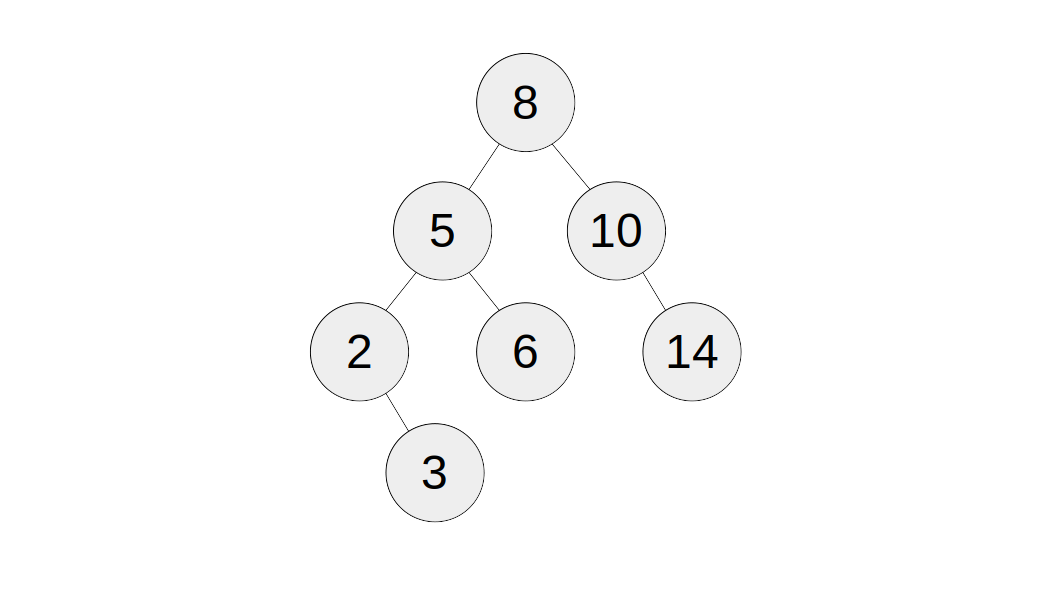# Closest BST Values II

Given a BST, output the `k` closest values in this BST to `x`. Sort the output by value.

The output set is guaranteed to be unique.

Do not convert the BST to a list.

### Input

• `bst`: a valid BST of size `n`.
• `x`: an integer representing the number to find the `k` closest numbers to.
• `k`: an integer.

### Output

A list of integers containing the `k` closest numbers to `x`.

### Examples

#### Example 1:

Input:

``````1bst = <See explanation>
2x = 7
3k = 4``````

Output: `[5, 6, 8, 10]`

Explanation:All four numbers in the output are within `3` away from `7`.

### Constraints

• `1 <= k <= n <= 10^5`